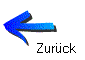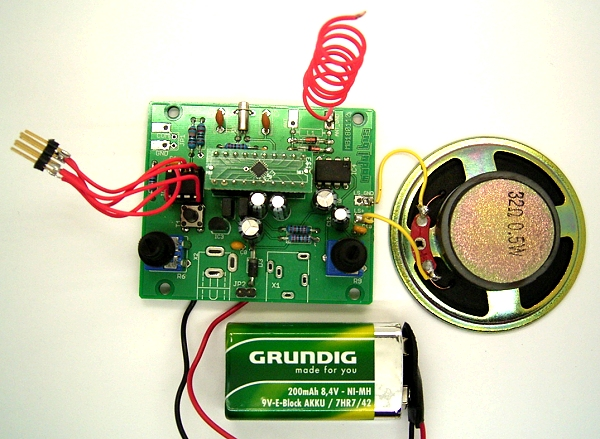#Dave asked me for help with a weather band receiver for use in the USA. He took  the Si4735Tiny13b BAS code on a ATtiny25 from ELEXS and changed it for a Si4736 FM-WB chip. The problem was to switch over from FM to WB and back. My first problem was that we don't use the NOAA Weather Radio in Germany. I found out that they use ten narrow band FM channels between 162.4MHz and 162.55MHz with 25 kHz between two channels.  It can be heard here: http://noaaweatherradio.org/

Dave used this code for WB:
`'Radio SI4735, UKW , Potiabstimmung\$regfile = "attiny25.dat"\$crystal = 1000000Dim F As WordDim H As ByteDim L As ByteDim Poti As WordDim Kanal As ByteDim Kanalalt As ByteConst F1 = 64980Const F2 = 64990Const F3 = 65000Const F4 = 65010Const F5 = 65020                                              'Need this oneDeclare Sub Freq Config Adc = Single , Prescaler = Auto Start Adc Config Scl = Portb.3 Config Sda = Portb.0 I2cinit Waitms 300 I2cstart I2cwbyte 34 I2cwbyte &H01 I2cwbyte &H13                                             '00 FM, 03 WB, 10 32-kHz-Osz I2cwbyte &H05 I2cstop Waitms 500 Kanalalt = 255 Do   Poti = Getadc(2)   Poti = Poti / 114                                       '0...8   If Poti = 0 Then Kanal = 0   If Poti = 2 Then Kanal = 1   If Poti = 4 Then Kanal = 2   If Poti = 6 Then Kanal = 3   If Poti = 8 Then Kanal = 4   If Kanal <> Kanalalt Then     If Kanal = 0 Then F = F1     If Kanal = 1 Then F = F2     If Kanal = 2 Then F = F3     If Kanal = 3 Then F = F4     If Kanal = 4 Then F = F5     Freq     Kanalalt = Kanal   End If LoopSub Freq   I2cstart   I2cwbyte 34   I2cwbyte &H50   I2cwbyte &H00   H = High(f)   L = Low(f)   I2cwbyte H   I2cwbyte L   I2cwbyte &H00   I2cstopEnd SubEnd `

The main difference to what I did for FM lies in the different initialization and in the different tuning. At first I was surprised to find constant frequencies in the range of 65000. Then I found this information in the SI documentation: Set the WB Receive to tune the frequency between 162.4MHz and 162.55MHz in 2.5kHz units. For example162.4MHz = 64960 and 162.55MHz = 65020.

So how can one switch between FM an WB? I remembered a similar situation, when I wanted to use AM and FM in the same software. The solution was to first send a Power Down command an then do a new initialization for FM mode or AM mode.

So I use the power_down command before every channel change. There are delays in these subs which still have to be tested out. What will be the shortest time to wait? This is the final version, working fine with FM and WB:

`'Radio SI4736, FM and WB  Pot tuning \$regfile = "attiny25.dat"\$hwstack = 16\$swstack = 16\$framesize = 16\$crystal = 1000000 Dim F As WordDim H As ByteDim L As ByteDim Poti As WordDim Kanal As ByteDim Kanalalt As Byte  Const F1 = 9230                                             'four FM channelsConst F2 = 9710Const F3 = 10190Const F4 = 10670Const F5 = 65020                                            'one WB channel = 162.550 mhz  Declare Sub Freq_wbDeclare Sub Freq_fmDeclare Sub Power_down();Declare Sub Init_wb()Declare Sub Init_fm() Config Adc = Single , Prescaler = AutoStart AdcConfig Scl = Portb.3Config Sda = Portb.0I2cinit Waitms 300  Kanalalt = 255Do   Poti = Getadc(2)   Poti = Poti / 114                                        '0...8   If Poti = 0 Then Kanal = 0   If Poti = 2 Then Kanal = 1   If Poti = 4 Then Kanal = 2   If Poti = 6 Then Kanal = 3   If Poti = 8 Then Kanal = 4   If Kanal <> Kanalalt Then     If Kanal = 0 Then F = F1     If Kanal = 1 Then F = F2     If Kanal = 2 Then F = F3     If Kanal = 3 Then F = F4     If Kanal = 4 Then F = F5     Power_down     If F > 20000 Then       Init_wb       Freq_wb      Else       Init_fm       Freq_fm     End If     Kanalalt = Kanal   End IfLoop Sub Freq_fm    I2cstart    I2cwbyte 34    I2cwbyte &H20    I2cwbyte &H00    H = High(f)    L = Low(f)    I2cwbyte H    I2cwbyte L    I2cwbyte &H00    I2cstopEnd Sub  Sub Freq_wb   I2cstart   I2cwbyte 34   I2cwbyte &H50   I2cwbyte &H00   H = High(f)   L = Low(f)   I2cwbyte H   I2cwbyte L   I2cwbyte &H00   I2cstopEnd Sub Sub Power_down  I2cstart  I2cwbyte 34  I2cwbyte &H11  I2cstop  Waitms 10End Sub Sub Init_wbWaitms 300I2cstartI2cwbyte 34I2cwbyte &H01I2cwbyte &H13                                              '00 FM, 03 WB,  10 32-kHz-OszI2cwbyte &H05I2cstopWaitms 500End Sub Sub Init_fm  Waitms 300  I2cstart  I2cwbyte 34  I2cwbyte &H01  I2cwbyte &H10                                             ' FM,  I2cwbyte &H05  I2cstop  Waitms 500End Sub  End`